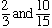Subject: Equivalent fractions please explain to us how to find the answer to this problem, we need three fractions that name the same amount as 2 Hi, Look at an example of two equivalent fractions first.are equivalent fractions. To see this, look at the second fraction, 10/15, and divide the numerator and denominator by 5. You get the first fraction. I could also see the equivalence in the other direction. That is start with the first fraction, 2/3, and MULTIPLY the numerator and denomintor by 5 to get the second fraction. The important point is that if you multiply or divide the numerator and denominator of a fraction by the same amount, you get an equivalent fraction. For your problem think of the number 2 as the fraction 2/1. Now multiply the numerator and denominator by the same amount, say 3. This gives the equivalent fraction 6/3. Just one more point. Above I should have said "if you multiply or divide the numerator and denominator of a fraction by the same NON-ZERO amount you get an equivalent fraction". If you multiply the numerator and denominator by zero you get 0/0 which is a meaningless quantity. Cheers, Penny Go to Math Central